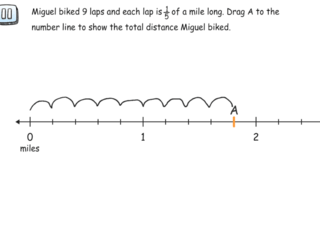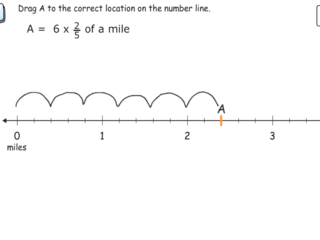# Interactive Problem Bank

•Fraction Circles
•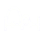Number Lines
•Fraction Bars
•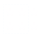Sets
•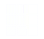Area Models
•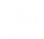Base Ten Blocks

## Multiplication of Fractions

Students solve word problems involving the multiplication of a fraction by a whole number using fraction circles, sets, real-world contexts, and number line.

### Multiplication of a Fraction by a Whole Number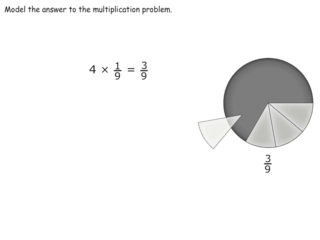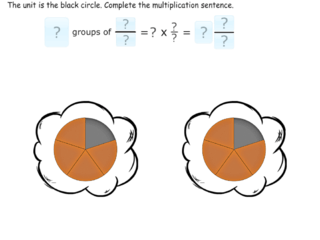### Multiplication of a Whole Number by a Fraction Using Sets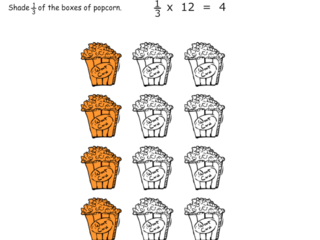### Multiplication of a Whole Number by a Fraction Using Number Line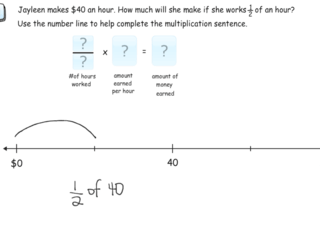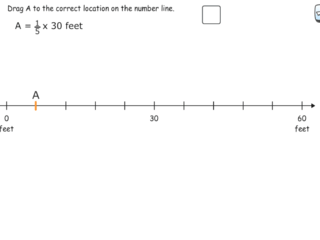### Multiplication of a Fraction by a Whole Number with Number Lines